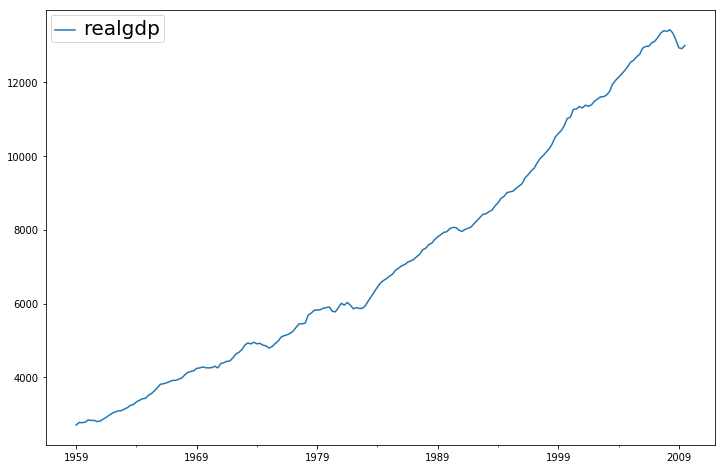/Statsmodels

# Time Series Filters

In :
%matplotlib inline

from __future__ import print_function
import pandas as pd
import matplotlib.pyplot as plt

import statsmodels.api as sm

In :
dta = sm.datasets.macrodata.load_pandas().data

In :
index = pd.Index(sm.tsa.datetools.dates_from_range('1959Q1', '2009Q3'))
print(index)

DatetimeIndex(['1959-03-31', '1959-06-30', '1959-09-30', '1959-12-31',
'1960-03-31', '1960-06-30', '1960-09-30', '1960-12-31',
'1961-03-31', '1961-06-30',
...
'2007-06-30', '2007-09-30', '2007-12-31', '2008-03-31',
'2008-06-30', '2008-09-30', '2008-12-31', '2009-03-31',
'2009-06-30', '2009-09-30'],
dtype='datetime64[ns]', length=203, freq=None)

In :
dta.index = index
del dta['year']
del dta['quarter']

In :
print(sm.datasets.macrodata.NOTE)

::
Number of Observations - 203

Number of Variables - 14

Variable name definitions::

year      - 1959q1 - 2009q3
quarter   - 1-4
realgdp   - Real gross domestic product (Bil. of chained 2005 US$, seasonally adjusted annual rate) realcons - Real personal consumption expenditures (Bil. of chained 2005 US$, seasonally adjusted annual rate)
realinv   - Real gross private domestic investment (Bil. of chained
2005 US$, seasonally adjusted annual rate) realgovt - Real federal consumption expenditures & gross investment (Bil. of chained 2005 US$, seasonally adjusted annual rate)
realdpi   - Real private disposable income (Bil. of chained 2005
US$, seasonally adjusted annual rate) cpi - End of the quarter consumer price index for all urban consumers: all items (1982-84 = 100, seasonally adjusted). m1 - End of the quarter M1 nominal money stock (Seasonally adjusted) tbilrate - Quarterly monthly average of the monthly 3-month treasury bill: secondary market rate unemp - Seasonally adjusted unemployment rate (%) pop - End of the quarter total population: all ages incl. armed forces over seas infl - Inflation rate (ln(cpi_{t}/cpi_{t-1}) * 400) realint - Real interest rate (tbilrate - infl)  In : print(dta.head(10))   realgdp realcons realinv realgovt realdpi cpi m1 \ 1959-03-31 2710.349 1707.4 286.898 470.045 1886.9 28.98 139.7 1959-06-30 2778.801 1733.7 310.859 481.301 1919.7 29.15 141.7 1959-09-30 2775.488 1751.8 289.226 491.260 1916.4 29.35 140.5 1959-12-31 2785.204 1753.7 299.356 484.052 1931.3 29.37 140.0 1960-03-31 2847.699 1770.5 331.722 462.199 1955.5 29.54 139.6 1960-06-30 2834.390 1792.9 298.152 460.400 1966.1 29.55 140.2 1960-09-30 2839.022 1785.8 296.375 474.676 1967.8 29.75 140.9 1960-12-31 2802.616 1788.2 259.764 476.434 1966.6 29.84 141.1 1961-03-31 2819.264 1787.7 266.405 475.854 1984.5 29.81 142.1 1961-06-30 2872.005 1814.3 286.246 480.328 2014.4 29.92 142.9 tbilrate unemp pop infl realint 1959-03-31 2.82 5.8 177.146 0.00 0.00 1959-06-30 3.08 5.1 177.830 2.34 0.74 1959-09-30 3.82 5.3 178.657 2.74 1.09 1959-12-31 4.33 5.6 179.386 0.27 4.06 1960-03-31 3.50 5.2 180.007 2.31 1.19 1960-06-30 2.68 5.2 180.671 0.14 2.55 1960-09-30 2.36 5.6 181.528 2.70 -0.34 1960-12-31 2.29 6.3 182.287 1.21 1.08 1961-03-31 2.37 6.8 182.992 -0.40 2.77 1961-06-30 2.29 7.0 183.691 1.47 0.81  In : fig = plt.figure(figsize=(12,8)) ax = fig.add_subplot(111) dta.realgdp.plot(ax=ax); legend = ax.legend(loc = 'upper left'); legend.prop.set_size(20);### Hodrick-Prescott Filter The Hodrick-Prescott filter separates a time-series$y_t$into a trend$\tau_t$and a cyclical component$\zeta_t$$$y_t = \tau_t + \zeta_t$$ The components are determined by minimizing the following quadratic loss function $$\min_{\\{ \tau_{t}\\} }\sum_{t}^{T}\zeta_{t}^{2}+\lambda\sum_{t=1}^{T}\left[\left(\tau_{t}-\tau_{t-1}\right)-\left(\tau_{t-1}-\tau_{t-2}\right)\right]^{2}$$ In : gdp_cycle, gdp_trend = sm.tsa.filters.hpfilter(dta.realgdp)  In : gdp_decomp = dta[['realgdp']].copy() gdp_decomp["cycle"] = gdp_cycle gdp_decomp["trend"] = gdp_trend  In : fig = plt.figure(figsize=(12,8)) ax = fig.add_subplot(111) gdp_decomp[["realgdp", "trend"]]["2000-03-31":].plot(ax=ax, fontsize=16); legend = ax.get_legend() legend.prop.set_size(20);### Baxter-King approximate band-pass filter: Inflation and Unemployment #### Explore the hypothesis that inflation and unemployment are counter-cyclical. The Baxter-King filter is intended to explictly deal with the periodicty of the business cycle. By applying their band-pass filter to a series, they produce a new series that does not contain fluctuations at higher or lower than those of the business cycle. Specifically, the BK filter takes the form of a symmetric moving average $$y_{t}^{*}=\sum_{k=-K}^{k=K}a_ky_{t-k}$$ where$a_{-k}=a_k$and$\sum_{k=-k}^{K}a_k=0$to eliminate any trend in the series and render it stationary if the series is I(1) or I(2). For completeness, the filter weights are determined as follows $$a_{j} = B_{j}+\theta\text{ for }j=0,\pm1,\pm2,\dots,\pm K$$ $$B_{0} = \frac{\left(\omega_{2}-\omega_{1}\right)}{\pi}$$ $$B_{j} = \frac{1}{\pi j}\left(\sin\left(\omega_{2}j\right)-\sin\left(\omega_{1}j\right)\right)\text{ for }j=0,\pm1,\pm2,\dots,\pm K$$ where$\theta$is a normalizing constant such that the weights sum to zero. $$\theta=\frac{-\sum_{j=-K^{K}b_{j}}}{2K+1}$$ $$\omega_{1}=\frac{2\pi}{P_{H}}$$ $$\omega_{2}=\frac{2\pi}{P_{L}}$$$P_L$and$P_H$are the periodicity of the low and high cut-off frequencies. Following Burns and Mitchell's work on US business cycles which suggests cycles last from 1.5 to 8 years, we use$P_L=6$and$P_H=32$by default. In : bk_cycles = sm.tsa.filters.bkfilter(dta[["infl","unemp"]])  • We lose K observations on both ends. It is suggested to use K=12 for quarterly data. In : fig = plt.figure(figsize=(12,10)) ax = fig.add_subplot(111) bk_cycles.plot(ax=ax, style=['r--', 'b-']);### Christiano-Fitzgerald approximate band-pass filter: Inflation and Unemployment The Christiano-Fitzgerald filter is a generalization of BK and can thus also be seen as weighted moving average. However, the CF filter is asymmetric about$t$as well as using the entire series. The implementation of their filter involves the calculations of the weights in $$y_{t}^{*}=B_{0}y_{t}+B_{1}y_{t+1}+\dots+B_{T-1-t}y_{T-1}+\tilde B_{T-t}y_{T}+B_{1}y_{t-1}+\dots+B_{t-2}y_{2}+\tilde B_{t-1}y_{1}$$ for$t=3,4,...,T-2$, where $$B_{j} = \frac{\sin(jb)-\sin(ja)}{\pi j},j\geq1$$ $$B_{0} = \frac{b-a}{\pi},a=\frac{2\pi}{P_{u}},b=\frac{2\pi}{P_{L}}$$$\tilde B_{T-t}$and$\tilde B_{t-1}$are linear functions of the$B_{j}$'s, and the values for$t=1,2,T-1,$and$T$are also calculated in much the same way.$P_{U}$and$P_{L}\$ are as described above with the same interpretation.

The CF filter is appropriate for series that may follow a random walk.

In :
print(sm.tsa.stattools.adfuller(dta['unemp'])[:3])

(-2.536458467334637, 0.10685366457233464, 9)

In :
print(sm.tsa.stattools.adfuller(dta['infl'])[:3])

(-3.0545144962572373, 0.030107620863485774, 2)

In :
cf_cycles, cf_trend = sm.tsa.filters.cffilter(dta[["infl","unemp"]])

                infl     unemp
1959-03-31  0.237927 -0.216867
1959-06-30  0.770007 -0.343779
1959-09-30  1.177736 -0.511024
1959-12-31  1.256754 -0.686967
1960-03-31  0.972128 -0.770793
1960-06-30  0.491889 -0.640601
1960-09-30  0.070189 -0.249741
1960-12-31 -0.130432  0.301545
1961-03-31 -0.134155  0.788992
1961-06-30 -0.092073  0.985356

In :
fig = plt.figure(figsize=(14,10))